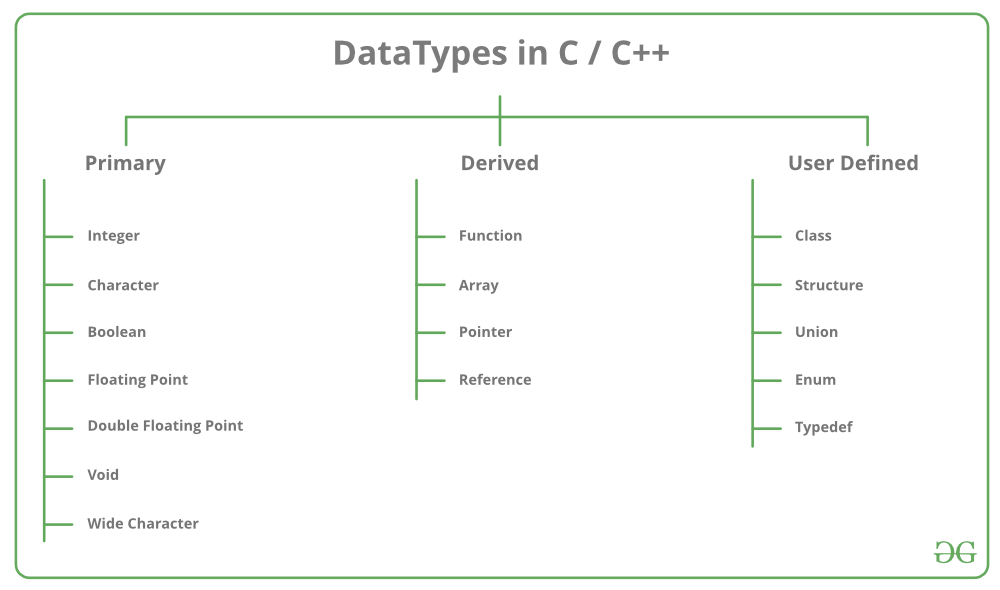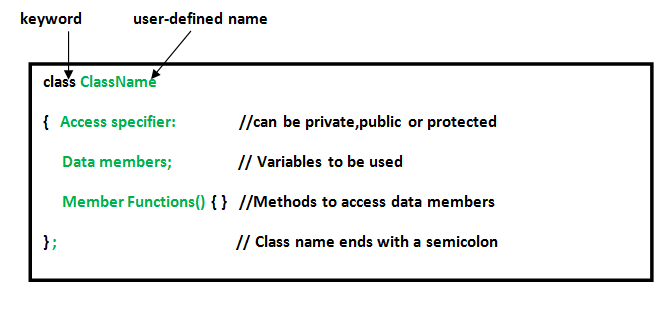# User defined Data Types in C++

Data types are means to identify the type of data and associated operations of handling it. There are three types of data types:

1. Pre-defined DataTypes
2. Derived Data Types
3. User-defined DataTypes### User-Defined DataTypes:

The data types that are defined by the user are called the derived datatype or user-defined derived data type.
These types include:

Below is the detailed description of the following types:

1. Class: The building block of C++ that leads to Object-Oriented programming is a Class. It is a user-defined data type, which holds its own data members and member functions, which can be accessed and used by creating an instance of that class. A class is like a blueprint for an object.

Syntax:Example:

 `// C++ program to demonstrate ` `// Class ` ` `  `#include ` `using` `namespace` `std; ` ` `  `class` `Geeks { ` `    ``// Access specifier ` `public``: ` `    ``// Data Members ` `    ``string geekname; ` ` `  `    ``// Member Functions() ` `    ``void` `printname() ` `    ``{ ` `        ``cout << ``"Geekname is: "` `<< geekname; ` `    ``} ` `}; ` ` `  `int` `main() ` `{ ` ` `  `    ``// Declare an object of class geeks ` `    ``Geeks obj1; ` ` `  `    ``// accessing data member ` `    ``obj1.geekname = ``"GeeksForGeeks"``; ` ` `  `    ``// accessing member function ` `    ``obj1.printname(); ` ` `  `    ``return` `0; ` `} `

Output:

```Geekname is: GeeksForGeeks
```
2. Structure: A structure is a user defined data type in C/C++. A structure creates a data type that can be used to group items of possibly different types into a single type.

Syntax:

```struct address {
char name;
char street;
char city;
char state;
int pin;
};```

Example:

 `// C++ program to demonstrate ` `// Structures in C++ ` ` `  `#include ` `using` `namespace` `std; ` ` `  `struct` `Point { ` `    ``int` `x, y; ` `}; ` ` `  `int` `main() ` `{ ` `    ``// Create an array of structures ` `    ``struct` `Point arr; ` ` `  `    ``// Access array members ` `    ``arr.x = 10; ` `    ``arr.y = 20; ` ` `  `    ``cout << arr.x << ``", "` `<< arr.y; ` ` `  `    ``return` `0; ` `} `

Output:

```10, 20
```
3. Union: Like Structures, union is a user defined data type. In union, all members share the same memory location. For example in the following C program, both x and y share the same location. If we change x, we can see the changes being reflected in y.

 `#include ` `using` `namespace` `std; ` ` `  `// Declaration of union is same as the structures ` `union` `test { ` `    ``int` `x, y; ` `}; ` ` `  `int` `main() ` `{ ` `    ``// A union variable t ` `    ``union` `test t; ` ` `  `    ``// t.y also gets value 2 ` `    ``t.x = 2; ` ` `  `    ``cout << ``"After making x = 2:"` `         ``<< endl ` `         ``<< ``"x = "` `<< t.x ` `         ``<< ``", y = "` `<< t.y ` `         ``<< endl; ` ` `  `    ``// t.x is also updated to 10 ` `    ``t.y = 10; ` ` `  `    ``cout << ``"After making Y = 10:"` `         ``<< endl ` `         ``<< ``"x = "` `<< t.x ` `         ``<< ``", y = "` `<< t.y ` `         ``<< endl; ` ` `  `    ``return` `0; ` `} `

Output:

```After making x = 2:
x = 2, y = 2
After making Y = 10:
x = 10, y = 10
```
4. Enumeration: Enumeration (or enum) is a user defined data type in C. It is mainly used to assign names to integral constants, the names make a program easy to read and maintain.

Syntax:

`enum State {Working = 1, Failed = 0}; `

 `// Program to demonstrate working ` `// of enum in C++ ` ` `  `#include ` `using` `namespace` `std; ` ` `  `enum` `week { Mon, ` `            ``Tue, ` `            ``Wed, ` `            ``Thur, ` `            ``Fri, ` `            ``Sat, ` `            ``Sun }; ` ` `  `int` `main() ` `{ ` `    ``enum` `week day; ` ` `  `    ``day = Wed; ` ` `  `    ``cout << day; ` ` `  `    ``return` `0; ` `} `

Output:

```2
```
5. Typedef : C++ allows you to define explicitly new data type names by using the keyword typedef. Using typedef does not actually create a new data class, rather it defines a name for an existing type. This can increase the portability(the ability of a program to be used across different types of machines; i.e., mini, mainframe, micro, etc; without much changes into the code)of a program as only the typedef statements would have to be changed. Using typedef one can also aid in self-documenting code by allowing descriptive names for the standard data types.

Syntax:

`typedef type name;`

where type is any C++ data type and name is the new name for this data type.
This defines another name for the standard type of C++.

Example:

 `// C++ program to demonstrate typedef ` `#include ` `using` `namespace` `std; ` ` `  `// After this line BYTE can be used ` `// in place of unsigned char ` `typedef` `unsigned ``char` `BYTE``; ` ` `  `int` `main() ` `{ ` `    ``BYTE` `b1, b2; ` `    ``b1 = ``'c'``; ` `    ``cout << ``" "` `<< b1; ` `    ``return` `0; ` `} `

Output:

```c
```
6. Rated as one of the most sought after skills in the industry, own the basics of coding with our C++ STL Course and master the very concepts by intense problem-solving.

My Personal Notes arrow_drop_upCheck out this Author's contributed articles.

If you like GeeksforGeeks and would like to contribute, you can also write an article using contribute.geeksforgeeks.org or mail your article to contribute@geeksforgeeks.org. See your article appearing on the GeeksforGeeks main page and help other Geeks.

Please Improve this article if you find anything incorrect by clicking on the "Improve Article" button below.

Article Tags :
Practice Tags :

11

Please write to us at contribute@geeksforgeeks.org to report any issue with the above content.